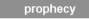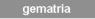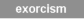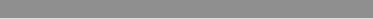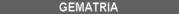In the very first verse of the Bible (Genesis 1:1), God sets the tone for gematria that continues richly throughout both the Hebrew Old Testament and Greek New Testament.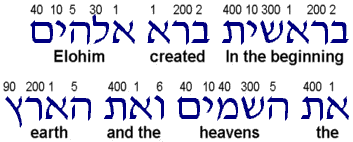"In the beginning God created the heavens and the earth." In Hebrew, this verse has seven words and 28 (7 × 4) letters. The number seven is repeated throughout the Bible as a number of perfection and completeness. For instance, there are seven feasts of Israel, seven good years and seven famine years interpreted in Pharaoh's dreams, seven years of service for each of Jacob's wives, seven lamps of the Menorah, seven trumpets, seven days' marching around Jericho, seven washings in the Jordan for Naaman, seven churches, seven candlesticks, seven seals, seven horns, seven eyes, seven stars, seven thunders, seven crowns, seven sprinklings of blood before the veil, seven Spirits of God, etc.

Ivan Panin, who wrote more than 42,000 pages of Bible gematria notes, found over 50 multiples of seven in the very first verse of the Bible. These multiples could not be due to chance. Above each letter in the graphic is the gematria or number value for that letter. By adding the numbers in different combinations, one can see many statistically meaningful multiples of the number seven. Here are just a few of the multiples:

1. The seven words in the first verse of the Bible have exactly 28 (7 × 4) letters.
2. The gematria of the first and last letters of the first half of the verse is 42 (7 × 6).
3. The gematria value of the first and last letters of the second half is 91 (7 × 13).
4. The nouns have 14 letters (7 × 2).
5. The sum of the nouns (God, heavens and earth) is 777 (7 × 111).
6. The place value of these nouns is 147 (7 × 21).
7. The Hebrew verb created has a value of 203 (7 × 29).
8. The first 3 words contain the subject and have exactly 14 letters.
9. The place value of these letters is 140 (7 × 20).
10. Of these 14, the place value of the silent letters is 28 (7 × 4).
11. And the place value of the non-silent letters is 112 (7 × 16).
12. The odd numbers among the 14 total 42 (7 × 6).
13. Even numbers among the 14 total 98 (7 × 14).
14. Multiplying the 14 place values of letters by their order is 1,008 (7 × 144).
15. The last four words contain the object and also have exactly 14 letters.
16. The object The Heavens has seven letters, as does the object and the earth.
17. The numeric value of the first and last letters of all words is 1,393 (7 × 199).
18. The sum of the first and last letters of the first and last words is 497 (7 × 71).
19. The value of the first and the last letters of each word between is 896 (7 × 128).
20. The sum of the factors of 896 is 21 (7 × 3).
21. The first letters of the first and last words have a gematria of 7.
22. The gematria of last letters of the first and last words is 490 (7 × 70).
23. The middle word and the one before it have together 7 letters.
24. The middle word and the one after it have together 7 letters.
25. The value of the first, middle, and last letters in the sentence is 133 (7 × 19).
26. Figures in the sum of God (8 + 6) total 14 (7 × 2).
27. Figures of the letters in God (1, 30, 5, 10, 40) also total 14 (7 × 2).
28. The sum of the place value figures of letters (1, 12, 5, 10, 13) is 14 (7 × 2).
29. The number of these place-value figures is 7.
30. Because of contractions in Hebrew (similar to the English isn't), the vocabulary of Gen. 1:1 is larger than the seven words of the verse. The nine-word vocabulary of Gen. 1:1 has a numeric value of 2275 (7 × 325).
31. The place value of the vocabulary is 259 (7 × 37).

The total gematria value for all seven words is 2701 (37 × 73). The figurate number 2701 is the 73rd triangle number, (73² +73)/2 = 2701. To signify His triune nature, God introduces a large triangle number. The First Person of the Holy Trinity, the Father, is represented by a star number. The figurate number 37, as a star number, takes the shape of a Star of David or Magen David (hexagram). In Hebrew, Magen David literally means Shield of David. God Himself was David's Shield (II Sam. 22:1-3). From 1 to 3313, there are only 24 star numbers, as this formula shows: 6n² –6n +1. Because 73 is also a star number, 2701 is the product of two star numbers. One can think of 2701 as a hexagram of hexagrams, since each counter making up the shape of the hexagram is itself a hexagram. Having octagonal and other symmetries, the prime number 37 is highly symmetrical. There are exactly 37 asterisks in each below example of 37 as the 4th centered hexagon, (3n² –3n +1) = 37, the 3rd star, (6n² –6n +1) = 37, and the 2nd truncated square, (7n² +4n +1) = 37:

```
*
* * * *            * *               * * *
* * * * *      * * * * * * *        * * * * *
* * * * * *      * * * * * *       * * * * * * *
* * * * * * *      * * * * *        * * * * * * *
* * * * * *      * * * * * *       * * * * * * *
* * * * *      * * * * * * *        * * * * *
* * * *            * *               * * *
*
```

Adding the seven Hebrew words of Genesis 1:1 in different combinations, one will find 23 multiples of the number 37, including a compound figurate square of hexagrams (37 × 7²) and cubes of hexagrams (37 × 2³) and (37 × 3³). Random chance would indicate only about three multiples. Note that each of the seven words is used exactly twelve times in making up the 23 multiples of 37 (i.e., 913 appears twelve times; 203 appears twelve times, and so on). Here are the gematria and order of each of the seven Genesis 1:1 words: 913, 203, 86, 401, 395, 407, 296.

1. 296 = 37 × 8
2. 407 = 37 × 11
3. 86 + 395 = 37 × 13
4. 407 + 296 = 37 × 19
5. 86 + 395 + 296 = 37 × 21
6. 86 + 395 + 407 = 37 × 24
7. 913 + 86 = 37 × 27
8. 203 + 401 + 395 = 37 × 27
9. 86 + 395 + 407 + 296 = 37 × 32
10. 203 + 401 + 395 + 296 = 37 × 35
11. 913 + 86 + 296 = 37 × 35
12. 913 + 86 + 407 = 37 × 38
13. 203 + 401 + 395 + 407 = 37 × 38
14. 913 + 203 + 401 = 37 × 41
15. 913 + 86 + 407 + 296 = 37 × 46
16. 203 + 401 + 395 + 407 + 296 = 37 × 46
17. 913 + 203 + 401 + 296 = 37 × 49
18. 913 + 203 + 401 + 407 = 37 × 52
19. 913 + 203 + 86 + 401 + 395 = 37 × 54
20. 913 + 203 + 401 + 407 + 296 = 37 × 60
21. 913 + 203 + 86 + 401 + 395 + 296 = 37 × 62
22. 913 + 203 + 86 + 401 + 395 + 407 = 37 × 65
23. 913 + 203 + 86 + 401 + 395 + 407 + 296 = 37 × 73

As though the complexity of encoding over fifty 7's and twenty-three 37's into the seven words of Genesis 1:1 were not enough, God has also encoded both two- and three-dimensional geometrical shapes. Adding the seven words in different combinations will produce statistically significant numbers of figurate polygons and polyhedra.

In the "select a sum" menu, the first three words of the Bible (913 + 203 + 86) have a gematria sum of 1202, the 10th points on the surface of a stella octangula, (12 × 10² +2) = 1202. The sum of the factors of 2701 (37 × 73) is 110, the 3rd points on the surface of a stella octangula (12 × 3² +2) = 110. Also note that 913 – 203 + 86 – 401 + 395 – 407 + 296 = 679, the 7th stella octangula n(2n² –1). The stella octangula is a three-dimensional version of a two-dimensional hexagram. If rotated just right in a shadow, it becomes a perfect Star of David. Two tetrahedrons fused into each other and offset 180 degrees, it is the only polyhedron that is perfectly inscribed in a cube. Here is an example of a stella octangula inscribed in a cube: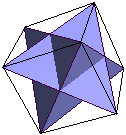Figurate numbers extend beyond the first verse. For instance, the total gematria value of the first eight words is 3003, the number 3003 being the 77th triangle number, (77² +77)/2 = 3003. From the second verse (Gen. 1:2) are the words, "And the Spirit of God moved upon the face of the waters." The total gematria value of this sentence is 1369 (37 × 37), a squared star number. One can also think of 1369 as the compound figurate shape of a hexagram of hexagrams. Genesis 1:4, the 4th verse of the Bible, has a gematria total of 1776 (37 × 48).

Just as He gave exact measurements for the Tabernacle, God also gave precise specifications for garments that the Levite priests wore. While ministering to God in holy office, the chief priest wore an ephod in finely embroidered blue, purple and red. Over his heart, he wore the Breastplate of Judgment as a memorial before God. The names of the twelve tribes of Israel were engraved in carbuncle, topaz, sardius, diamond, sapphire, emerald, amethyst, agate, ligure, jasper, onyx and beryl (Ex. 28:17-20). Set in gold filigree, the gems were in four rows and three columns. Below are the order and gematria value for each name.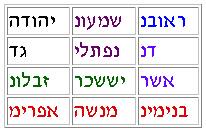Judah 30 Simeon 466 Reuben 259 Gad 7 Naphtali 570 Dan 54 Zebulun 95 Issachar 830 Asher 501 Ephraim 331 Manasseh 395 Benjamin 162

This list of names matches God's list of tribes (Num. 2:1-32). Note that Levi, as a priest tribe, is excluded from being numbered (Num. 1:49, 2:33).

When added, each of the six color matched groups is divisible by 37. The sum of all twelve names is 3700, a square of hexagrams (37 × 10²). The figurate number 3700 is also the 43rd points on the surface of a tetrahedron (2 × 43² +2 = 3700). One may think of a tetrahedron as a three-dimensional triangle pointing to the triune nature of God. The sum of the factors of 3700 (2 × 2 × 5 × 5 × 37) is 51, the 3rd stella octangula n(2n² –1), a three dimensional Star of David. Reading right to left from the top, the sum of the odd numbered names is 1850, exactly one-half of 3700. The sum of the even numbered names is 1850 as well. The gematria of the bottom row, all in red, is 888 (37 × 8 × 3), the Greek gematria value of Jesus (Ιησους) or (iota 10, eta 8, sigma 200, omicron 70, upsilon 400 and sigma 200). The number eight is associated with Jesus because, among other things, it represents salvation and a new covenant in the Bible. For instance, there were eight people saved in Noah's ark. Isaac was circumcised on the eighth day, etc. The sum of the green and purple names is 1480 (37 × 8 × 5), the gematria of Christ (Χριστος) or (chi 600, rho 100, iota 10, sigma 200, tau 300, omicron 70 and sigma 200). The "8" association with Messiah is deeper, as the numerical sum of vowels in Jesus is 488 (8 × 61), and that of Christ, 80 (8 × 10). The sum of consonant letters in Jesus is 400 (8 × 50), and that of Christ, 1400 (8 × 175). Other Greek names for Messiah are also divisible by eight. Lord (Κυριος) has a value of 800. Truth (Αληθεια) is 64 (8 × 8). Savior (Σωτηρ) has a value of 1408 (8 × 8 × 22). Alpha and the Omega (Α και Ω) is 832 (8 × 8 × 13). Messenger (Αγγελος) is 312 (8 × 39). Messiah (Μεσσιας) is 656 (8 × 82). Way (Οδος) has a value of 344 (8 × 43).

The "8" association with Messiah and the "37" Star of David relationship are seen by adding name values in the Breastplate. One finds the following multiples of 296 (8 × 37). Note that the multiples of 296 are in perfect order (1 × 296, 2 × 296, 3 × 296, 4 × 296, 5 × 296, 6 × 296, 7 × 296, 8 × 296 and 9 × 296). Every name below is used exactly five times to make the multiples of 296.

• 259 + 30 + 7 = 1 × 296
• 30 + 54 + 7 + 501 = 2 × 296
• 162 + 395 + 331 = 3 × 296
• 259 + 30 + 7 + 162 + 395 + 331 = 4 × 296
• 54 + 501 + 830 + 95 = 5 × 296
• 259 + 30 + 54 + 7 + 501 + 830 + 95 = 6 × 296
• 259 + 830 + 95 + 162 + 395 + 331 = 7 × 296
• 54 + 501 + 830 + 95 + 162 + 395 + 331 = 8 × 296
• 259 + 30 + 54 + 7 + 501 + 830 + 95 + 162 + 395 + 331 = 9 × 296

Here are visual representations of Breastplate values.

The gematria of Jesus (888) plus that of Christ (1480) is 2368 (37 × 4³), a cube of hexagrams. Subtracting 2368 from the Breastplate total (3700) leaves 1332 (6² × 37), a square of hexagrams. Subtracting 1332 from the Genesis 1:1 total (2701) leaves 1369 (37 × 37), a hexagram of hexagrams. 2701 minus 888 is 1813 (7² × 37), a square of hexagrams, and 2701 minus 2368 is 333 (3² × 37), another square of hexagrams.

-----Proverbs 25:2
It is the glory of God to conceal a thing: but the honour of kings is to search out a matter.

The last word of the Bible is amen, or αμην in its original Greek. The Greek gematria value of αμην is 99 (alpha 1, mu 40, eta 8, nu 50). Gematria of the first word (913) of the Bible plus that of the last word (99) is 1012 (2² × 253), a square of hexagrams. The figurate number 253 is the 7th star number, (6n² –6n +1) = 253. It is also the 22nd triangle number, (22² +22)/2 = 253. The place values of these first and last words of the Bible total 109 (76 + 33), the 9th centered triangle, (3n² -3n +2)/2 = 109. Gematria of the first letter (bet 2) of the Bible plus that of the last (nu 50) forms a three-dimensional triangle, a tetrahedron, since 52 is the 5th points on the surface of a tetrahedron (2 × 5² +2) = 52. The last word of the Hebrew Bible is ויעל (and he shall go up). Its gematria, 116 (lamed, ayin, yod, vav), plus that of the first word (913) of the Bible is 1029, a cube of triangles (7³ × 3). The place values of these two words total 120 (76 + 44), also a triangle number, (15² + 15)/2 = 120.

Psalm 117, the shortest chapter of the Bible, has a gematria of 2294 (37 × 62). Psalm 119, the longest chapter of the Bible, has gematria of 332,617, a number 50 short of 332,667 (999 × 333). Since 50 is the value of the letter nun, this missing nun fits the missing-letter pattern of other Psalms. For instance, the 23 verses of Psalm 34 start with every letter of the Hebrew alphabet except vav. The 40 verses of Psalm 37 start with every letter except ayin, and the 21 verses of Psalm 145 start with every letter except nun. The number 332,667 is very interesting, since 999 (37 × 3³) and 333 (37 × 3²) are rich in figurate multiples within multiples.

Underscoring the Holy Trinity are both two- and three-dimensional triangle numbers. Adding together the Hebrew gematria values of God (YHWH 26 heh, vav, heh, yod, or יהוה), Jesus (Yeshua 386 ayin, vav, shin, yod, or ישוע) and Holy Spirit (Ruach ha'Kodesh 623 shin, dalet, qoph, heh, chet, vav, resh, or רוחהקדש) is 1035. Besides being the 11th centered tetrahedron, (2 × 11 +1)(11² + 11 + 3)/3 = 1035, the figurate number 1035 is the 45th triangle, (45² +45)/2 = 1035. The sum of the factors of 1035 (3 × 3 × 5 × 23) is 34, the 4th points on the surface of a tetrahedron (2 × n² +2). Hebrew place values of YHWH, Yeshua and Ruach ha'Kodesh total 162 (26 + 53 + 83), a cube of triangles (3³ × 6).

Additionally, the gematria value of YHWH is the 2nd points on the surface of a cube (6 × 2² +2 = 26). The gematria value of Yeshua is the 8th points on the surface of a cube (6 × 8² +2 = 386), while the figurate number 296 is the 7th points on the surface of a cube (6 × 7² +2 = 296). The most holy place in both the Tabernacle and the Temple, THE HOLY OF HOLIES, is a cube (II Chr. 3:8; I Kings 6:20). In Daniel 8:13, Messiah appears as a Christophany called Palmoni, פלמוני, a name some Bibles annotate as "numberer of secrets" or "wonderful numberer," (since that is what Palmoni means in Hebrew). The Hebrew letters for Palmoni (yod, nun, vav, mem, lamed, peh) have a gematria of 216, a cube (6³). The sum of the factors of 216 (2 × 2 × 2 × 3 × 3 × 3) is 15, both the 5th triangle number (n² +n)/2, and the 2nd centered tetrahedral number (2n³ + 3n² + 7n +3)/3. The number 216 is the perimeter value of both the 73rd triangle (2701) and the 19th star (2053). The place value of Palmoni (72) is also the perimeter value of both the 25th triangle (325) and the 7th star (253).

The Hebrew word Israel (lamed, aleph, resh, shin, yod, or ישראל) has a gematria of 541, the 10th star number (6n² –6n +1). In the star are 37 rows of counters. The place value of Israel is 64 (12 + 1 + 20 + 21 + 10), the 4th cube (4³ = 64), and the 7th centered triangle (3n² –3n +2)/2 = 64. Note that the gematria of Jesus Christ (2368) is 37 × 64.

An interesting observation about the Bible is that if a number is mentioned in the text, one may find the same number encoded in the text. For instance, the passage starting at John 21:11, in which Christ's disciples caught exactly 153 fish, various words associated with fishing have gematria divisible by 153: fishes (ιχθυες) has a value of 153 × 8, and the net (το δικτυον) has the same 153 × 8 value. The figurate number 153 is the 17th triangle number, (17² +17)/2 = 153. It is a truncated triangle number, too, as 1 and 15 are also triangle numbers. The reverse of 153 (351) is the 26th triangle number, (26² +26)/2 = 351. In the Bible are various triangle numbers, such as the days of creation (6), the number of God's Commandments (10), the number of books in the Bible (66), the disciples in the Upper Room (120), the number saved in Paul's shipwreck (276), the length of Noah's Ark (300 cubits), and the number of man (666). Of course, all of these triangle numbers point to the triune nature of God.

The base Trinity number is three. Gematria of the third word of the Bible, Elohim, אלהים, a plural form of God, dramatically underscores the Holy Trinity. Gematria values of the five Hebrew letters of Elohim (mem, yod, heh, lamed, aleph) total 86 (40, 10, 5, 30, 1). Note that these values can be read as three groups of three, i.e. (5 + 30 + 1), (10 + 5 + 30) and (40 + 10 + 5). Sums of these groups are 36, 45 and 55, a rising sequence of consecutive triangle numbers (8th, 9th and 10th). These three triangles total 136, itself a triangle number. In the 22 letters of the Hebrew alphabet, what are the odds that a five-letter sequence has three groups of consecutive triangle numbers? Of the 5,153,632 possible combinations (22 × 22 × 22 × 22 × 22) there are only 42 such groupings. Only one of them is a valid Hebrew word: Elohim.

If we look at the first 17 verses of Matthew, a gospel dealing with the genealogy of Jesus Christ, we note that it contains 72 Greek vocabulary words. We find the following hepatic (7) structure throughout these original Greek versus. Note that everything is divisible by seven. What are the odds that all of the Bible's multiples of seven, 37, etc., are chance? The odds are less than one out of googolplex. Not even Microsoft's Bill Gates, with all of his money, could hire mathematicians sharp enough to encode even seven Hebrew words with the number of multiples in Genesis 1:1, let alone encode the number of pages in the average Bible (about 900 pages).

1. The number of words which are nouns is exactly 56, or 7 × 8.
2. The Greek word the occurs exactly 56 times, or 7 × 8.
3. The occurs in exactly 7 different forms.
4. There are two main sections in the passage: verse 1-11 and 12-17. In the first main section, the number of Greek vocabulary words used is 49, or 7 × 7.
5. The sum of the factors of 49 is 14, or 7 × 2.
6. Of these 49 words, The number of those beginning with a vowel is 28, or 7 × 4.
7. The number of words beginning with a consonant is 21, or 7 × 3.
8. The number of words ending with a vowel is 7 (7 × 1).
9. The number of words ending with a consonant is 42 (7 × 6).
10. The total number of letters in these 49 words is exactly 266, or 7 × 38.
11. The sum of the digits in 266 is 14 (7 × 2).
12. The sum of the factors of 266 (7 × 2 × 19) is 28 (7 × 4).
13. The numbers of vowels among these 266 letters is 140, or 7 × 20.
14. The number of consonants is exactly 126, or 7 × 18.
15. Of these 49 words, the number of words that occur more than once is 35, or 7 × 5.
16. The number of words occurring only once is 14, or 7 × 2.
17. The number of words that occur in only one form is exactly 42, or 7 × 6.
18. The number of words appearing in more than one form is also 7.
19. Of the 49 Greek vocabulary words, 42, or 7 × 6 are nouns.
20. The number of words that are not nouns is 7.
21. Of the nouns, 35 are proper names, or 7 × 5.
22. These 35 nouns are used 63 times, or 7 × 9.
23. The number of male names is 28, or 7 × 4.
24. These male names occur 56 times or 7 × 8.
25. The number that are not male names is 7.
26. Three women are mentioned: Tamar, Rahab, and Ruth. The number of Greek letters in these three names is 14, or 7 × 2.
27. The number of compound nouns is 7.
28. The number of Greek letters in these 7 nouns is 49, or 7 × 7.
29. Only one city is named in this passage, Babylon, which in Greek contains exactly 7 letters.

Here is just a partial listing of the multiples of seven in Matthew 1:18-25, an account of Christ's Virgin Birth:

1. The number of Greek words in the passage is exactly 161 (23 × 7).
2. The number of Greek letters in the 161 words is 896 (128 × 7).
3. The numeric value of these, or gematria, is exactly 93,394 (13,342 × 7).
4. The number of vocabulary words used is 77 (11 × 7); their gematria is 52,605 (7,515 × 7).
5. 6 Greek words are used here which are found nowhere else in Matthew. Their value is 5,005 (715 × 7).
6. These 6 Greek words have 56 letters (8 × 7).
7. The 161 words occur in 105 forms (15 × 7); their numeric value is 65,429 (9,347 × 7).
8. In the 105 forms there are 35 verbs (5 × 7).
9. There are exactly 7 proper names.
10. The number of letters in these is 42 (6 × 7).
11. One word, Emmanuel, is used nowhere else in the New Testament; its gematria is 644 (92 × 7). The sum of its figures is 14 (2 × 7).
12. The number of forms exclusive to this passage is 14 (2 × 7); their numeric value is 8,715 (1,245 × 7).
13. In speaking to Joseph, the angel uses of the 77 vocabulary words exactly 28 (4 × 7).
14. The numeric value of all his words is exactly 21,042 (3,006 × 7).
15. The angel uses 35 different forms (5 × 7); these forms have exactly 168 letters (24 × 7).
16. The numeric value of these letters is 19,397 (2,771 × 7).
17. In using 28 of the vocabulary words the angel leaves exactly 49 (7 × 7).
18. In using exactly 35 forms he leaves exactly 70 (10 × 7).
19. The sum of the digits in 70 is 7; its factors (7 × 2 × 5) added equal 14 (2 × 7)

Because the authenticity of Mark 16: 9-20 had been questioned, the passage had not been included in some Bibles. Ivan Panin showed, through the richness and continuity of its gematria, that the passage belongs. Gematria, then, is a type of watermark or seal of authenticity. Because the Apocrypha, Book of Mormon, Qur'an, etc., do not have rich gematria multiples, we know that they are not from God. Below are some of the 75 features of seven Ivan Panin found in these twelve verses.

1. The number of words in this passage is 175 (7 × 25).
2. The vocabulary has 98 words (7 × 14).
3. The number of its forms is 133 (7 × 19).
4. The numeric value of its 133 forms is 89,663 (7 × 12,809).
5. Of these 133 forms 112 (7 × 16) occur but once; and 21 (7 × 3) occur more than once.
6. The 98 words of the vocabulary have 553 letters (7 × 79).
7. Of which 294 (7 × 42) are vowels, and 259 (7 × 37) are consonants.
8. Of the 98 vocabulary words 84 (7 × 12) are found before in the Gospel of Mark, and 14 (7 × 2) are found only here.
9. Of the 98 vocabulary words 42 (7 × 6) are used by Messiah in His address to the disciples; and 56 (7 × 8) form no part of His vocabulary.
10. Messiah's speech has 56 (7 × 8) words, and the rest of the passage has 119 (7 × 17) words.
11. The vocabulary is not only seven, but of seven sevens (7 × 7 × 2).
12. The same is true of 294 (7 × 7 × 6).
13. Of 84 (7 × 2 × 2 × 3), itself a multiple of seven, and the sum of its factors, 14, (7 × 2).
14. The sum of the figures in 133 is 7.
15. The 21 forms occurring more than once have 231 letters (7 × 11 × 3), itself 33 sevens.
16. With the sum of its factors 21 (7 × 3).
17. The 175 words are distributed among three natural divisions in the narrative: verses 9-11 have 35 (7 × 5) words, verses 12-18 have 105 (7 × 15) words, and verses 19-20 have 35 (7 × 5) words.
18. The total numeric value of the letters of all the words in the passage is 103,663, or 14,809 × 7
19. The numeric value of verse 9 is 11,795 (7 × 1,685).
20. The numeric value of verse 10 is 5,418 (7 × 774).
21. The numeric value of verse 11 is 11,795 (7 × 1,685).
22. The numeric value of verses 12 to 20 is 86,450 (7 × 12,350).
23. The first word of verse 10 has a value of 98 (7 × 14), the middle word, 4,529 (7 × 647), and the last word, 791 (7 × 113).
24. The word deadly in verse 18 is not found elsewhere in the New Testament. With a numeric value of 581 (7 × 83), deadly is preceded in the vocabulary by 42 (7 × 8) words, and in the passage by 126 (7 × 18) words.

Another type of encoded evidence is known as equidistant letter sequences, or ELS. God uses ELS and gematria together. For instance, adding every seventh Hebrew letter of Isaiah 9:6, a well-known Messianic prophecy, totals 888, the gematria of Messiah (30 + 50 + 400 + 5 + 6 + 6 + 90 + 1 + 300).

-----Isaiah 9:6
For a child will be born to us, a son will be given to us; And the government will rest on His shoulders; And His name will be called Wonderful Counselor, Mighty God, Eternal Father, Prince of Peace.

Adding every seventh Hebrew letter of Isaiah 11:1, another Messianic prophecy, also totals 888 (200 + 10 + 200 + 5 + 10 + 6 + 40 + 200 + 3 + 8 + 1 + 5 + 200). Some people question why God would encode such complication into His writing. Well, you and I write in ways reflecting our limited intelligence. God, with His writing, reflects His unlimited intelligence. It would be unusual if God did not write in such a way. Is such a feat too difficult for the Creator of the Universe? Think about it.

The through-the-roof statistical probabilities of encoded figurate number patterns in the Bible could not possibly be there by random chance. One has better odds of sitting down at a poker table and being dealt a royal flush fourteen times in a row. By the way, the odds against this happening (one chance in 649,74014) is a number higher than the number of atoms in the known universe. The reason math evidence is so strong is that there are formal proofs for math. Only somebody who is illogical and mentally ill will try to reject math evidence. Agrarian Hebrews thousands of years ago did not have the math skills to encode such gematria patterns within patterns. Even mathematicians today cannot do it. Only God could have done it. God wrote the Bible. The math evidence of God is convincing to a rational, logical, and sane person.